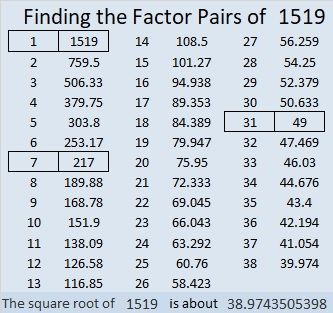# What Kind of Shape is 1519 in?

### Today’s Puzzle:

1519, 1520, and 1521 are all figurate numbers. What kind of shape can you arrange 1519 tiny dots?1519 is the 23rd centered hexagonal number because 23³ – 22³ = 1519.

It is also the 23rd centered hexagonal number because it is one more than six times the 23rd triangular number. Do you see the 23rd triangular number six times in the graphic above?

### Factors of 1519:• 1519 is a composite number.
• Prime factorization: 1519 = 7 × 7 × 31, which can be written 1519 = 7² × 31
• 1519 has at least one exponent greater than 1 in its prime factorization so √1519 can be simplified. Taking the factor pair from the factor pair table below with the largest square number factor, we get √1519 = (√49)(√31) = 7√31
• The exponents in the prime factorization are 2 and 1. Adding one to each exponent and multiplying we get (2 + 1)(1 + 1) = 3 × 2 = 6. Therefore 1519 has exactly 6 factors.
• The factors of 1519 are outlined with their factor pair partners in the graphic below.